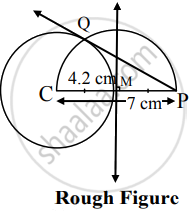# Draw a circle of radius 4.2 cm. Draw a tangent to the circle from a point 7 cm away from the center of the circle - Geometry

Diagram

Draw a circle of radius 4.2 cm. Draw a tangent to the circle from a point 7 cm away from the center of the circle

#### SolutionSteps of construction:

1. With centre C, draw a circle of radius 4.2 cm
2. Take point P such that CP = 7 cm.
3. Draw the perpendicular bisector of seg CP. It intersects CP in point M.
4. With M as centre and radius equal to CM, draw an arc intersecting the circle in points Q.
5. Draw ray PQ.
Ray PQ is the required tangent to the circle.
Concept: Construction of a Tangent to the Circle at a Point on the Circle
Is there an error in this question or solution?

Share